# Difference between revisions of "0708-1300/Class notes for Tuesday, November 27"

Announcements go here

## Today's Agenda

• The planimeter with a picture from http://whistleralley.com/planimeter/planimeter.htm but our very own plane geometry and Stokes' theorem.
• Completion of the proof of Stokes' theorem.
• Completion of the discussion of the two- and three-dimensional cases of Stokes' theorem.
• With luck, a discussion of de-Rham cohomology, homotopy invariance and Poincaré's lemma.

## Class Notes

The notes below are by the students and for the students. Hopefully they are useful, but they come with no guarantee of any kind.

### First Hour

Planimeter

A planimeter consists of two rods connected with a join where the end of one rod is fixed (but free to rotate) and the opposing end of the second rod traces out the boundary of some surface on the plane. I.e., the planimeter is kind of like a 1 legged roach. At the join of the two rods is a wheel which rotates (and measures the rotation) when the rod tracing the boundary moves in the normal direction and simply slides back an forth when moved in a tangential direction.

Now we recall from plane geometry that we can locate points in the polar form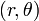$(r,\theta)$ and have the equations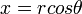$x = rcos\theta$ and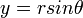$y = rsin\theta$

Hence,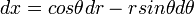$dx = cos\theta dr - rsin\theta d\theta$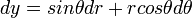$dy = sin\theta dr + rcos\theta d\theta$

Hence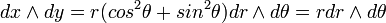$dx\wedge dy = r(cos^2\theta + sin^2\theta)dr\wedge d\theta = rdr\wedge d\theta$

Now, the planimeter is essentially a 1 form corresponding to the speed of the wheel. We consider a diagram where the angle from the horizontal at the fixed end of the planimeter to the measuring end is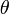$\theta$ and the angle from the horizontal to the first rod (the one connected to the fixed point) is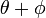$\theta + \phi$. Hence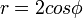$r = 2cos\phi$ and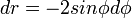$dr = -2sin\phi d\phi$

With a little plane geometry we can see that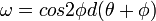$\omega = cos2\phi d(\theta + \phi)$

Computing,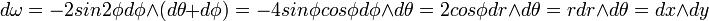$d\omega = -2 sin2\phi d\phi\wedge(d\theta + d\phi) = -4 sin\phi cos\phi d\phi\wedge d\theta = 2cos\phi dr\wedge d\theta = rdr\wedge d\theta = dx\wedge dy$

Now applying stokes theorem, the the planimeter integrates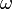$\omega$ over the boundary of our surface and hence this is just the integral of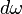$d\omega$ over the surface. But this is just the integral of the area form.

Hence the planimeter measure the area of a surface.

Back to Stokes Theorem

Firstly recall that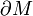$\partial M$ is oriented so that if you prepend the outward normal to its orientation you get the orientation of M

Alternatively we recall that neighborhoods of points on the boundary look like the half space. Hence we can choose to restrict our attention to atlas's where all charts look like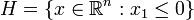$H= \{x\in\mathbb{R}^n:x_1\leq 0\}$

We can see that these orientations are the same, i.e., just prepend the outward normal to the half space.

Proof of Stokes

We have now defined all the terms. WLOG$\omega$ is supported in one chart (by linearity)

For a compactly supported n-1 form on H need to show that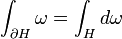$\int_{\partial H}\omega = \int_H d\omega$

We let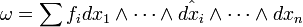$\omega = \sum f_i dx_1\wedge\cdots\wedge \hat{dx_i}\wedge\cdots\wedge dx_n$ (where the hat means it is omitted)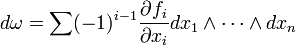$d\omega = \sum (-1)^{i-1} \frac{\partial f_i}{\partial x_i} dx_1\wedge\cdots\wedge dx_n$

So,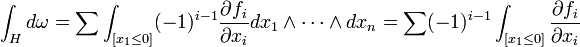$\int_H d\omega = \sum \int_{[x_1\leq 0]}(-1)^{i-1}\frac{\partial f_i}{\partial x_i}dx_1\wedge\cdots\wedge dx_n = \sum (-1)^{i-1}\int_{[x_1\leq 0]}\frac{\partial f_i}{\partial x_i}$

via fundamental theorem of calculus and that the f_i's are compactly supported we get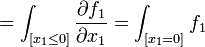$= \int_{[x_1\leq 0]} \frac{\partial f_1}{\partial x_1} = \int_{[x_1=0]} f_1$

Hence with the standard inclusion of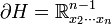$\partial H = \mathbb{R}^{n-1}_{x_2\cdots x_n}$ we get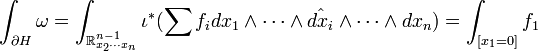$\int_{\partial H}\omega = \int_{\mathbb{R}^{n-1}_{x_2\cdots x_n}}\iota^*(\sum f_i dx_1\wedge\cdots\wedge \hat{dx_i}\wedge\cdots\wedge dx_n) = \int_{[x_1=0]}f_1$

Thus these are the same and the theorem is proved Q.E.D.

Real Plane

Consider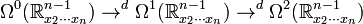$\Omega^0(\mathbb{R}^{n-1}_{x_2\cdots x_n})\rightarrow^d\Omega^1(\mathbb{R}^{n-1}_{x_2\cdots x_n})\rightarrow^d\Omega^2(\mathbb{R}^{n-1}_{x_2\cdots x_n})$

Forms in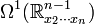$\Omega^1(\mathbb{R}^{n-1}_{x_2\cdots x_n})$ look like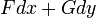$Fdx +Gdy$ and map under d to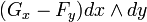$(G_x - F_y)dx\wedge dy$

Hence applying Stokes' Theorem: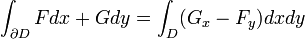$\int_{\partial D}Fdx + Gdy = \int_D (G_x-F_y)dxdy$

This is known as Greens Theorem

In complex analysis we also have a similar result Cauchy's Theorem where the integral of an analytic function around a closed path is zero. This is because analytic functions obey the Cauchy-Riemann equations and hence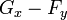$G_x-F_y$ is identically zero.

### Second Hour

Coming soon to a wikipedia near you.